ATMS 411 Homework [Main Page] [Daily Notes] [Final Project]

Homework style example.
Homework style example with a graph.

ONLINE ASSIGNMENTS ARE GIVEN IN WEBCAMPUS.

TEAMS FOR HOMEWORK ASSIGNMENTS WHEN REQUIRED
T3 The Too Tired. (Bowman, Broc, Gabriela)
Those Guys (Robert, Daniel, Palina)
Baklava (Michael, Gina)
Isothermal (Joseph, Shuang, Hatef)
FC Spartak Moscow (Jacob, Kevin, Penelope)
Gliding High (Kylara, Jingting, Nathan)
Group 7 (Kaitlin, Ryan O.)

Homework 5. Read chapter 4. The first two problems are related to climate and radiation issues.
1. Do problem 4.21.

2. A. Do problem 4.29.
B. Extra credit. Estimate also the radiative relaxation time for the Earth's oceans taken together as one body of water.

3. A. This problem uses the one dimensional radiation transfer equation we discussed in class. You may also want to read this article that describes the theory as well. At what optical depth do water droplet containing clouds have the greatest amount of diffuse solar radiation transmitted through them? [Hints: assume that cloud droplets all have a diameter of 17 microns, and use the Mie Theory Calculator to calculate the asymmetry parameter g at a wavelength of 550 nm. You'll need to use the refractive index of water at this wavelength. You can make a plot of diffuse radiation as a function of optical depth and read the peak off the graph, or use the Excel solver to obtain the optical depth.]

B. The image below shows the relative probability of obtaining different cloud optical thicknesses (same as cloud optical depth). Read the cloud optical depth off of the graph that corresponds with the peak counts. Calculate the cloud reflectance (albedo) at this optical depth when the cloud is above ground that is very dark (has an albedo of zero)? Calculate the cloud albedo when it is above ground with a surface albedo of 0.2.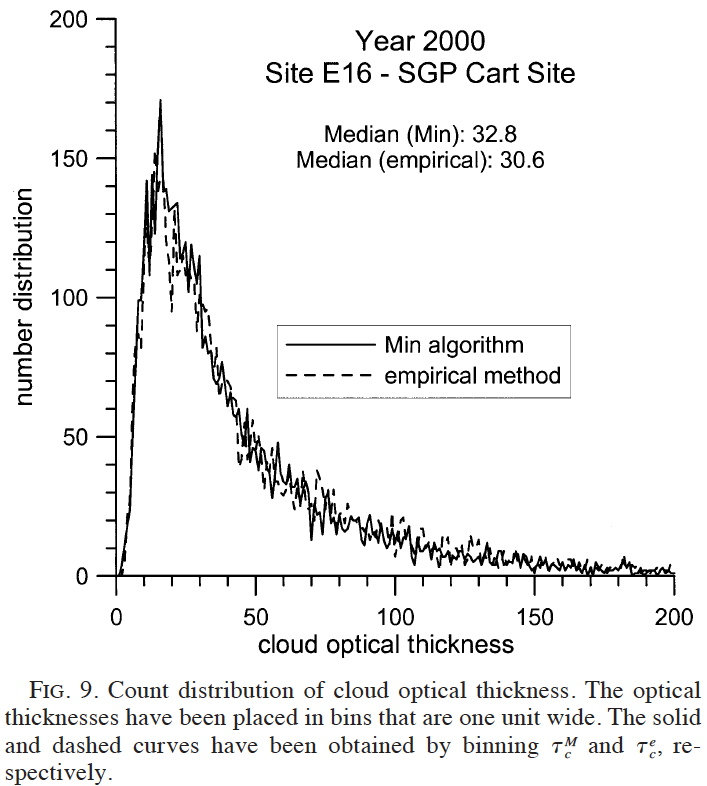Cloud optical depth from this reference. Click image for larger version

C. Extra credit. Fit the cloud optical thickness curve in part B to a log normal distribution function. From the fit parameters, calculate the average cloud albedo above a dark ground.

D. Second Extra Credit: Consider the top of atmosphere albedo under partly cloudy conditions. Assume that the surface albedo under clear skies is A=0.08. From your result in part C, obtain the cloud fraction fc implied by this model so that the combined albedo under partly cloudy conditions is Rtotal = 0.31.

4. This problem refers to the 1 layer atmosphere model we discussed in class, and is related to the idea of geo engineering --- the idea of putting stuff into the atmosphere to reduce the amount of sunlight at the surface, to cool the planet. Common discussions are about using scattering aerosol to geo engineer by placing them into the stratosphere. This problem considers a different idea, putting black carbon aerosol into the aerosol that primarily absorb light. We are actually doing a lot of this planet-wide, as a consequence of incomplete combustion, especially from diesel fueled vehicles and sources. We'll assume that the affect of aerosol can be modeled by the solar transmissivity τ used in the theory.
Use an atmospheric emissivity of ε=0.7 for this problem in parts A through C.

A. What are the surface and atmospheric temperatures when the solar transmissivity τ is equal to 1? Explain why the surface temperature in this case is higher than the surface temperature found for the astronomical problem when we considered only the outgoing infrared radiation budget (where we obtained a brightness temperature of 255 K).

B. Now adjust the solar transmissivity τ until the surface temperature is lowered by 1 Kelvin. What is the solar transmissivity that gives a reduction of surface temperature by 1 Kelvin? What is the corresponding atmosphere temperature? Comparing the atmospheric temperature difference between parts A and B, what is likely to happen with the atmosphere that changes temperature by the amount you find?

C. What mass concentration of black carbon aerosol, denoted by BC [units of grams/m3], is needed to obtain the solar transmissivity τ given in part B, assuming a mass absorption efficiency of MAE=10 square meters per gram, and that the aerosol is in a H=5000 meters thick layer (assume constant air density for this calculation). Is this mass concentration ever likely to be found in the atmosphere [convert your answer to micrograms/m3 units]? [Hint: solve for BC from the relationship for the direct beam, τ=exp(-BC*MAE*H].

D. Extra credit: Extend the 1 layer atmosphere model to 2 layers having an atmospheric boundary layer coupled to the surface and the free atmosphere above. Check that the model makes sense. Explore the model predictions for temperature of the two layers and the surface. By induction, can you see how to extend this model to a N-layer atmosphere? Answer the important question, does the surface warm or cool when the black carbon aerosol absorber is in the first layer, the second layer, or in equal amounts in each? You can choose an emissivity of the first layer equal to ε=0.7, and let the second layer have an emissivity of ε=0.6. Calculate the surface and layer temperatures as a function of the absorption coefficient.

5. A. What is the ratio of Rayleigh scattering strength for blue light compared with red light by gases in the atmosphere?
B. How does this affect the color of the sky?
C. Describe the polarization of light seen when looking at 90 degrees from the direction of the sun on a clear day (no clouds or aerosol, just air).

6. Do problem 4.56. This problem is intended to help you think about the Earth's radiation budget shown in Figures 4.34 and 4.35.

Homework 4: Group presentations and each person turns in the assignment.
Read chapter 4. Do problem 4.11. It has many parts, so start early.
It is given here in part 1 and part 2.
Note: There is an error/issue in the description of problem 4.11q.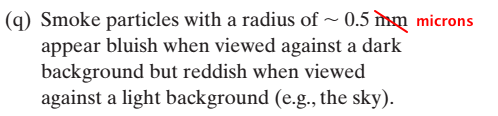Group presentations are as follows:

T3 The Too Tired. (w, x, y, z)
Those Guys (s, t, u, v)
Baklava (p, q, r)
Isothermal (l, m, n, o)
FC Spartak Moscow (h, i, j, k)
Gliding High (d, e, f, g)
Group 7 (a, b, c)

We will work on parts (aa, bb, cc) together.

Homework 3. Each team will do a report and a presentation for the following. (One report and presentation from each team).
Preparation for this homework includes our class discussions, the MetEd module, and you may read section 8.3.1a on Deep Convection, pg 344-347 of Wallace and Hobbs.

1. For each team member, find an exciting sounding from anywhere and anytime in the world (using the Univ. of Wyoming site) with a lot of convective available potential energy (CAPE).
Obtain both the GIF skew T image and the text file. We will use the text file for the sounding to do the calculations.

a. Identify the level of free convection and the equilibrium level. These can be read from the sounding indices, or at the intersections given by the lifted surface parcel and environmental air.

b. Using the sounding text, calculate the CAPE in units of Joules/kg, the estimated vertical velocity at the equilibrium level in meters/second and compare with the value on the sounding.
Also, calculate the precipitable water vapor amount in units of mm and compare with the values given on the sounding.
(Here's the another version; it also has the calculation of the moist adiabatic lapse rate.)

c. Make a graph of the vertical distribution of potential temperature (THTA in the sounding text) and equivalent potential temperature (THTE in the sounding text).
In your discussion, identify stable, unstable, neutrally stable, and convectively stable regions.
Here's an example; the top height is at just above the equilibrium level.
Plot the data for THTA and THTE in the sounding, not the model curves.

d. Obtain the 500 mb height map from reanalysis for your sounding for the time of the sounding, and including the location of your sounding.
Here is the specific link to use for obtaining it from reanalysis.
The latitude and longitude range for your graph can be centered at the balloon observation by finding the latitude and longtitude for it, and going +-20 degrees north and sound, and west and east for the bounding box.
Interpret in terms of locations of low and high heights, and wind speed maxima and minima.
Here's an example from the 28July2017 0z sounding location, Lamont Oklahoma located at about 36.7 North and 97.6 West: Settings and 500 mb plot. Lamont Oklahoma will be in about the center of the image.

Report:
In the report, each team member will be listed as a coauthor.
The goal is to make your report as short as possible, but to include everything.
Introduction: Discuss CAPE and precipitable water vapor amount in the introduction, and discuss the importance of each. Be sure to define each variable when using equations.

Section 1. Include the skewT graph from the Univ of Wyoming and its interpretation. One figure for each team member.

Section 2. Give a table of values for your locations and include values in your table for:
a. Equivalent potential temperature θE for your air parcel (from the spreadsheet). This value is constant for the entire trajectory of the air parcel lifted from the surface.
b. Lifting condensation level height and pressure.
c. Level of free convection in meters and pressure value for it.
d. Equilibrium level height and pressure.
e. Your calculated CAPE value in Joules/kg.
f. Your calculated vertical velocity in meters/second at the equilibrium level based on use of your CAPE value.
g. Your precipitable water amount in mm.
Use one table with data from all team members.

Section 3. Prepare one graph with all the potential temperature and equivalent potential temperature curves versus height for each team member, to compare them.
You may need to have one graph for potential temperature, and one for equivalent potential temperature if they get too busy to put all on a single graph.
Discuss atmospheric stability in terms of these graphs.

Section 4. Prepare one 500 mb level graph for each team member. Discuss.

Appendix: Optional extra credit. You may include any related atmospheric data (like archived radar data, maps of other pressure levels, etc) that helps tell your story.

Presentation:
Each team member will explain their own skewT graph.
One team member can do the section 2 discussion.

Tools and Extras
1. Soundings from the University of Wyoming site.
2. You may also obtain archived data for the 500 mb level, and precipitation data from radar imagery here.
3. Historical weather may be obtained here, mostly from surface stations at airports.

Homework 2. Students will turn in the assignment and present problems as assigned for their group.
Turn in problems through web campus.

Do problem 3.18. (Groups will be assigned parts of this problem to present in class.)
Do problems 3.20, 3.23, 3.26 (also discussed in class), 3.28.
(it will be helpful to read problems 3.21 and 3.22 before doing 3.23).

Note: On problem 3.28, if the energy release, 5x106 J/m2 is from the latent heat released in the formation of a liquid water cloud,
the cloud might have properties as follows: 100 m thick, 600 cloud droplets per cm3, 20 micron cloud radius, liquid water path 2 kg/m2,
since the latent heat release from cloud droplet condensation is 2.5x106 Joules/kg. For those interested, here's a journal article on cloud properties.
(From this information it is possible to calculate the cloud optical depth, albedo, and transmission).

Problem 3.18 Presentation Assignments (each person presents at least one problem).

a, b, c (The Too Tired)

d, e, f (Those guys)

g, h, i (Baklava)

j, k, l (Isothermal)

m, n, o (FC)

p, q, r (Gliding High)

s, t (Group 7)

u, v, w (Group 8)

Homework 1. Turn in this homework assignment through webCampus, using Microsoft Word.
A. Do problem 1.6. Write your answers into the first part of the MSword document you will be turning in for this assignment.

B. Do problem 1.12. Go to the South Pole and find a sounding that has a temperature structure like this. Include the SkewT LogP graph in your report.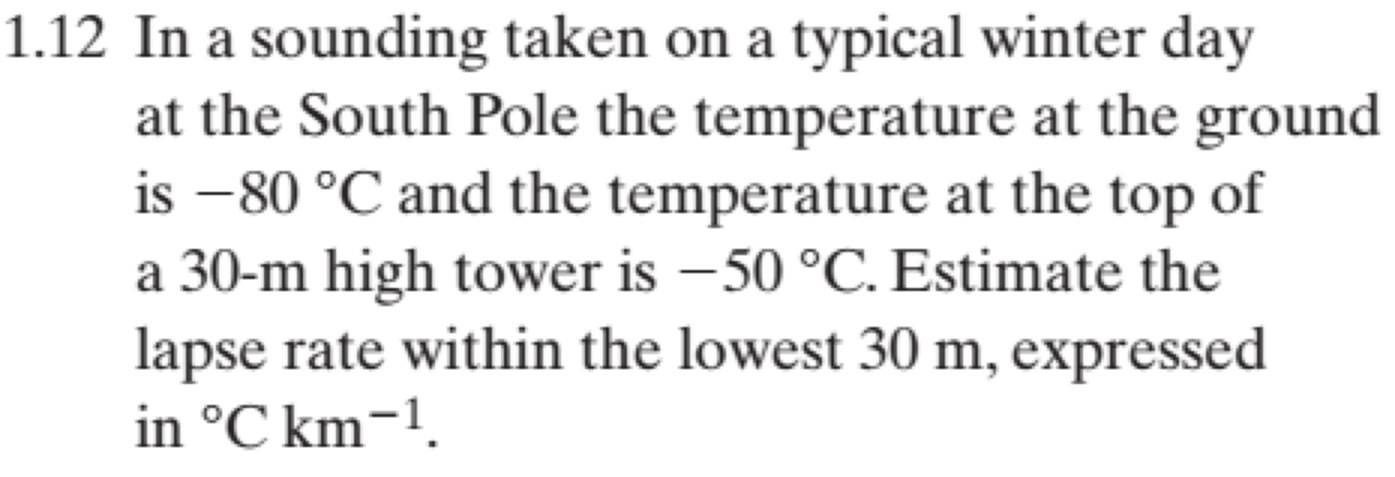C. Write a short report for the following analysis.
Calculate a time series of the average mass per unit area (units of kg / m2) of the air between UNR and DRI for the month of January 2018, and between DRI and Slide Mountain for the month of July 2018.
Calculate a time series of the lapse rate (-dT/dz) between these stations as well and overlay with the first graph.
You will have a total of 2 graphs, one for each month.
Discuss the variation of the average mass per unit area, and its relationship, if any, with the lapse rate, or other variables you might explore.

We will develop some theory in class for this problem.
The time series for the sites need to be synchronized, to take into account any missing data.
The UNR pressure sensor is not properly temperature compensated.
Here's what we use to compensate it for temperature variation
.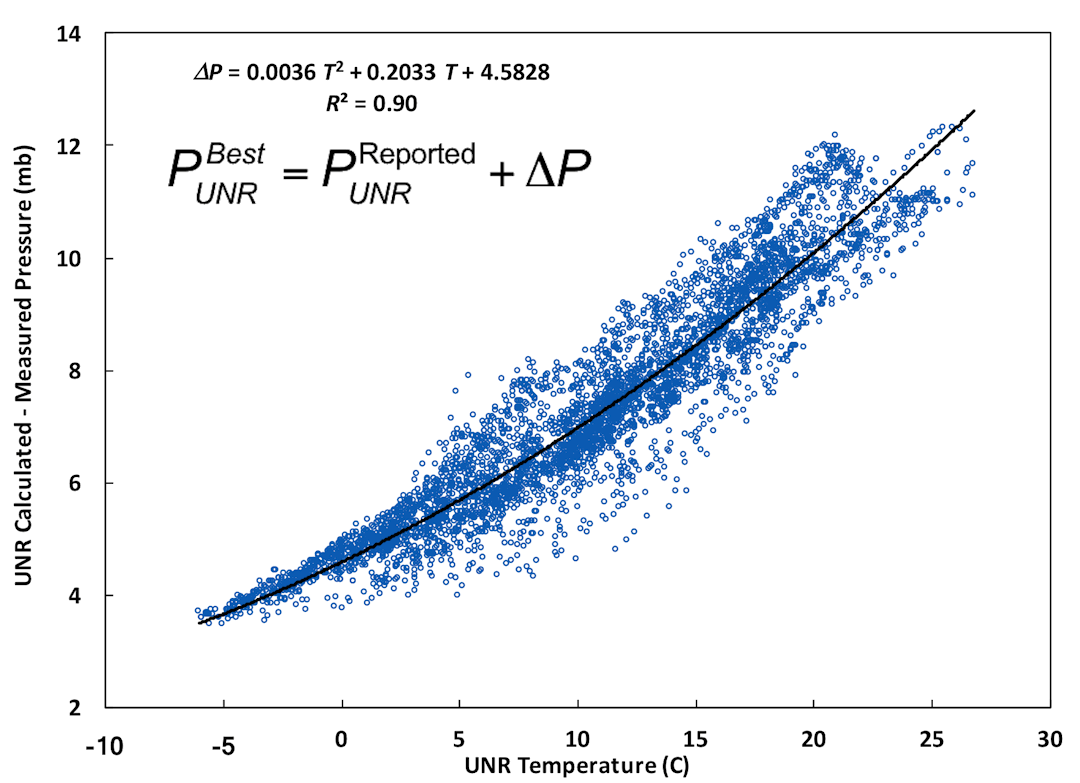Click on image for larger version and equation to use for compensating pressure.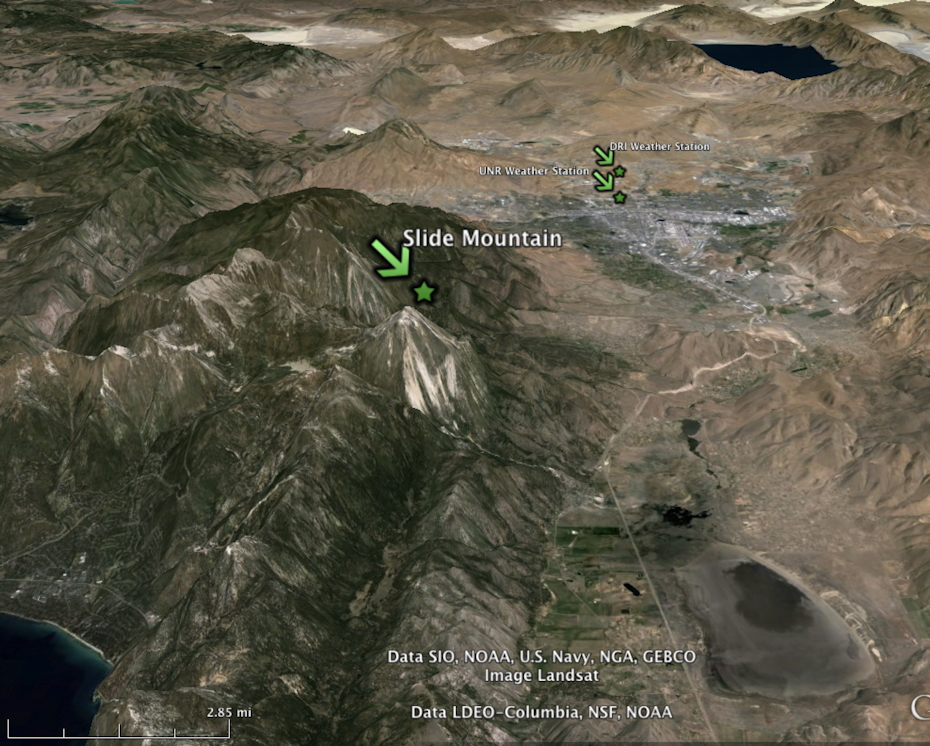Weather station images looking north. Lake Tahoe is on the lower left, Pyramid Lake on the upper right. The UNR weather station is on the valley floor. Click on image for larger version.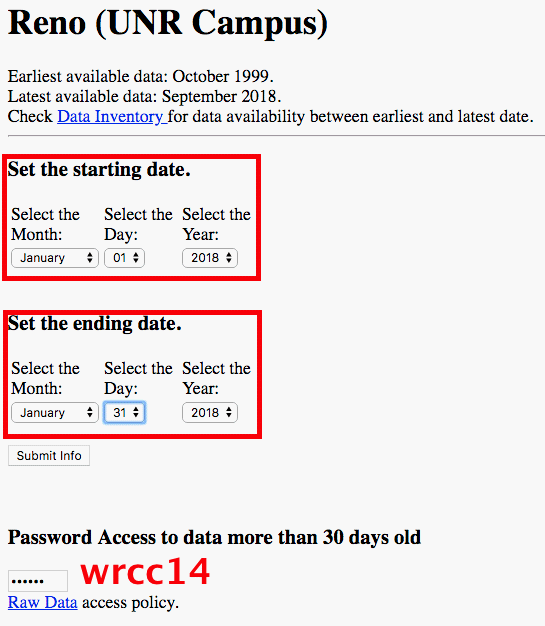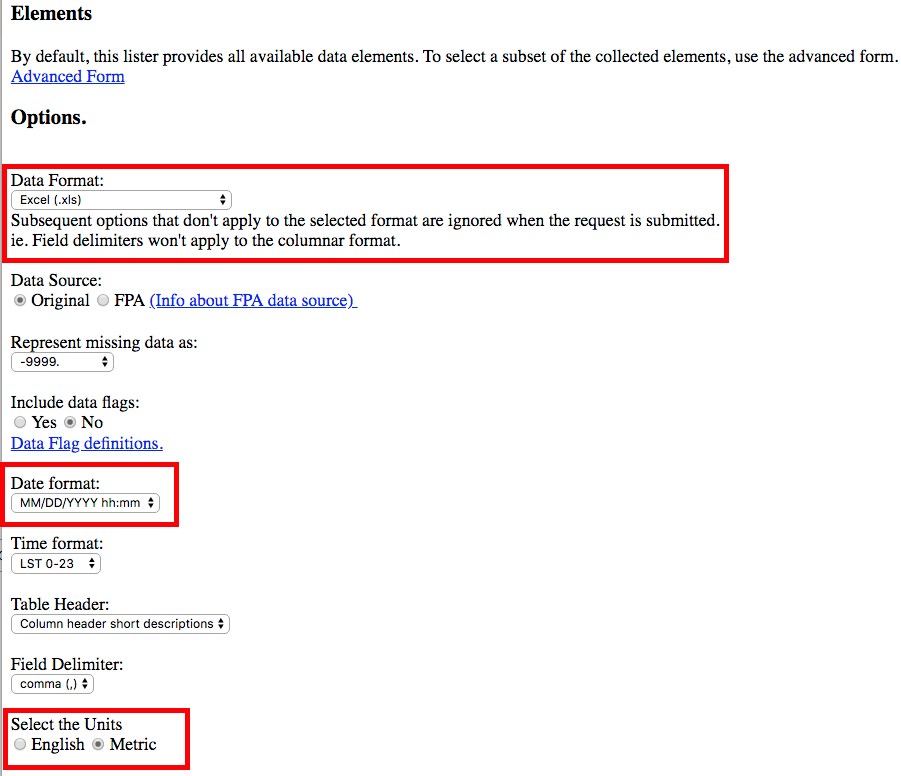NOTE: A short report should be written like a short section in a text book.
A. Title for the report. Your name.
B. First paragraph describes what's in the report, describes what is to be accomlished.
C. Provide figures, each figure with a number and caption. Figures must be in publication format -- high quality figures with 16 point (or greater) bold black font; tick marks inside. All axes 1 point thick and black.
D. Each figure must be discussed in the text by number, describing the significance of the figure and its relationship to other figures as needed.
E. Any equations should be offset, as in a textbook, and each equation should have a number. Refer to equations by number.
F. The last paragraph should summarize the overall outcome of the report.
Get started early.
Take advantage of the UNR writing center to have them read your report draft to give you feedback on writing quality.

TOOLS:
A. Meteorological data can be obtained from the University of Wyoming web site.
B. Most (or all) computers readily accessible to all students, using their netID, have Google Earth, MSword, and Excel.
C. You can use your netID to also access these software packages through the UNR remote services application.

FINAL PROJECT, START NOW!

Deliverables:
ATMS 411 in class presentation and turn in presentation here.
ATMS 611 in class presentation, report, and turn in presentation here.
Presentations are 5 to 10 minutes long.

Atmospheric Physics students take photographs of the atmosphere or environment, and explain the Atmospheric Physics connection.
For example, blue sky, sky polarization, coronas, halos, rainbows, lenticular clouds, gravity waves, lightning, water phase clouds, ice phase clouds,
inferring air motions and winds from cloud structures, contrails, vortices in contrails, sky color during pollution events, sky color near the horizon, sky color at sunset looking to the east.
Photographs of the dendritic nature of ice growing on windshields on cold days, the shape and nature of icicles, dew on a moist mornings are also possible topics.
Photographs of snow flakes and snow crystals, here's a discussion.
If you have special hobbies or work, like paragliding, Atmospheric Physics related aspects can be included in your project.
You can use soundings, satellite images, etc, to also help tell the story.

ATMS 411 students will do a presentation
ATMS 611 students will do a presentation and a report.

Resources that may help

Gravity wave discussion.
Snow crystal/flake observations.
Cloud identification.
NASA WorldView for satellite imagery. You can add layers for additional information.
National Weather Service balloon soundings, served by the Univ of Wyoming.